# PSAT Math : How to graph an ordered pair

## Example Questions

### Example Question #4 : How To Graph An Ordered Pair

Which of the following coordinate pairs is farthest from the origin?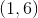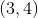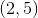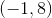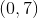Using the distance formula, calculate the distance from each of these points to the origin, (0, 0). While each answer choice has coordinates that add up to seven, (-1, 8) is the coordinate pair that produces the largest distance, namely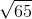, or approximately 8.06.